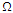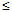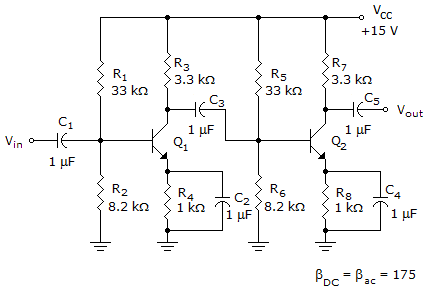# Electronic Devices - BJT Amplifiers

### Exercise :: BJT Amplifiers - General Questions

56.

Which of the following configurations has a voltage gain of –RC /re?

 A. Fixed-bias common-emitter B. Common-emitter voltage-divider with bypass capacitor C. Fixed-bias common-emitter and voltage-divider with bypass capacitor D. Common-emitter voltage-divider without bypass capacitor

Explanation:

No answer description available for this question. Let us discuss.

57.

An emitter-follower amplifier has an input impedance of 107 k. The input signal is 12 mV. The approximate output voltage is (common-collector)

 A. 8.92 V B. 112 mV C. 12 mV D. 8.9 mV

Explanation:

No answer description available for this question. Let us discuss.

58.

Which of the following is (are) true regarding the output impedance for frequencies in the midrange100 kHz of a BJT transistor amplifier?

 A. The output impedance is purely resistive. B. It varies from a few ohms to more than 2 M. C. An ohmmeter cannot be used to measure the small-signal ac output impedance. D. All of the above

Explanation:

No answer description available for this question. Let us discuss.

59.

Refer to this figure. The output signal from the first stage of this amplifier is 0 V. The trouble could be caused byA. an open C4. B. an open C2. C. an open base-emitter of Q1. D. a shorted C4.

Explanation:

No answer description available for this question. Let us discuss.

60.

What is the limit of the efficiency defined by = Po / Pi?

 A. Greater than 1 B. Less than 1 C. Always 1 D. None of the above